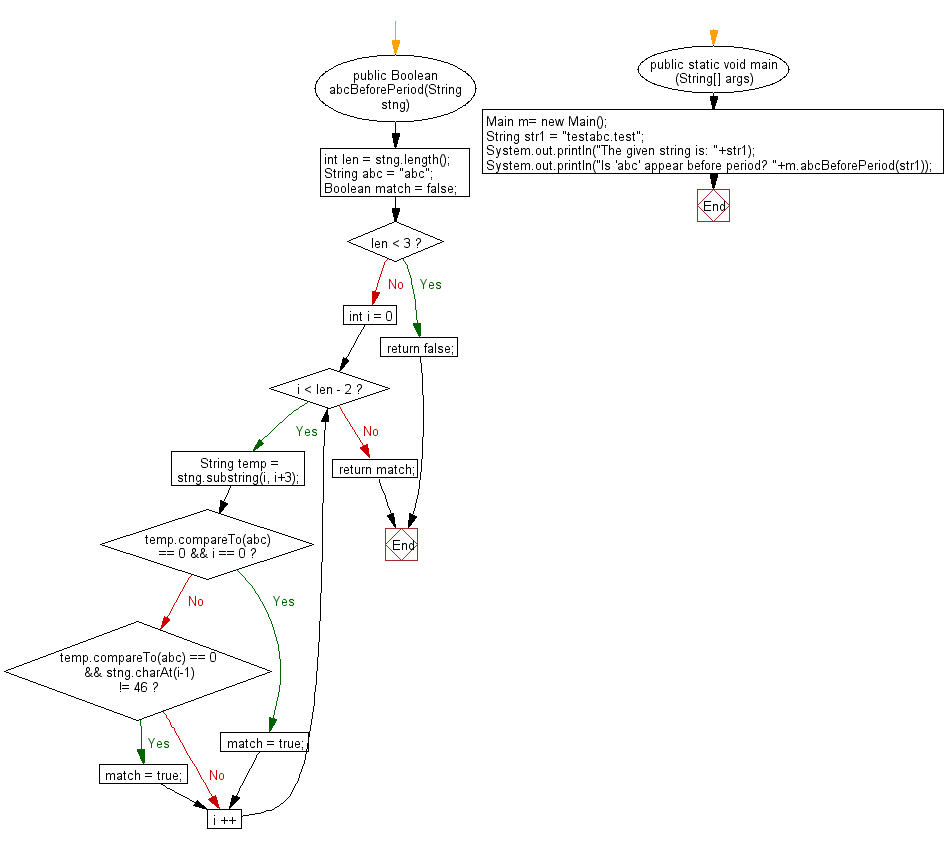﻿ Java exercises: Return true if the given string contains an appearance of 'abc' but not directly a period(.) - w3resource# Java String Exercises: Return true if the given string contains an appearance of 'abc' but not directly a period(.)

## Java String: Exercise-73 with Solution

Write a Java program to return true if the given string contains an appearance of 'abc' but not directly a period(.)and followed by.

Sample Solution:

Java Code:

``````import java.util.*;
public class Main
{
public Boolean abcBeforePeriod(String stng)
{
int len = stng.length();
String abc = "abc";
Boolean match = false;
if (len < 3)
return false;
for (int i = 0; i < len - 2; i ++)
{
String temp = stng.substring(i, i+3);
if (temp.compareTo(abc) == 0 && i == 0)
match = true;
else if(temp.compareTo(abc) == 0 && stng.charAt(i-1) != 46)
match = true;
}
return match;
}
public static void main (String[] args)
{
Main m= new Main();
String str1 =  "testabc.test";
System.out.println("The given string is: "+str1);
System.out.println("Is 'abc' appear before period? "+m.abcBeforePeriod(str1));
}
}
``````

Sample Output:

```The given strings is: testabc.test
Is 'abc' appear before period? true

The given string is: test.abctest
Is 'abc' appear before period? false
```

Pictorial Presentation:Flowchart:Java Code Editor:

Improve this sample solution and post your code through Disqus

What is the difficulty level of this exercise?

﻿